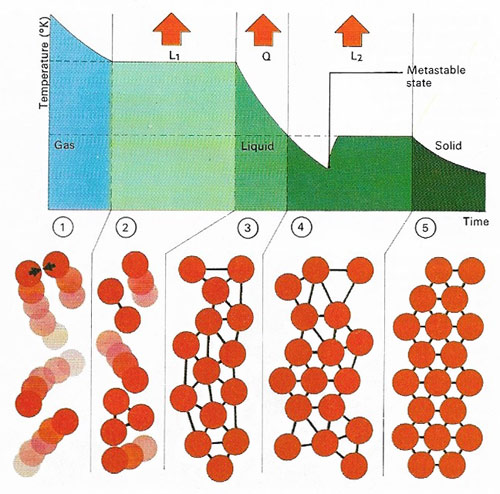Akinetic theory of gasesVibrations of atoms and molecules in a substance governs its temperature. In a gas (1) atoms move independently and their average velocity and mass determines the internal energy and temperature. After cooling, loss of latent heat of condensation (L1) converts the gas at the boiling-point to a liquid state (2) when its atoms become locked in a weakly bonded arrangement. Further cooling (3) to the freezing-point loses quantity of heat (Q) and a solid then forms through (4) release of latent heat of fusion (L2); the atoms then become rigidly bonded together (5). A colder 'metastable' phase can precede actual freezing.

The kinetic theory of gases is a mathematical explanation of the behavior of gases based on the model that gases consist of molecules (or atoms) in constant motion in space, the kinetic energy of the molecules depending on the temperature of the gas. The kinetic theory makes seven assumptions:

1. The volume occupied by the gas molecules themselves is negligible compared with the volume of space between them.
2. All the particles that make up the gas are identical.
3. The distribution of energy among particles is random.
4. There are sufficient numbers of molecules for the statistical average to be meaningful.
5. Collisions are all perfectly elastic.
6. The molecules travel in straight lines between collisions.
7. Newtonian mechanics can be applied to molecule interactions.

The pressure exerted by a gas on the walls of the vessel is due to the collision of the molecules with it. The gas laws may be shown to be in full agreement with this theory.